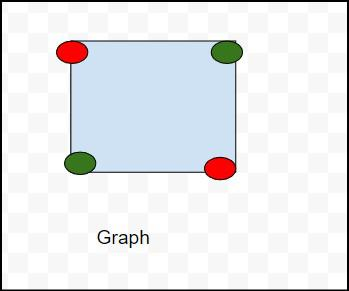Open In App
Related Articles
• Write an Interview Experience
• Discrete Mathematics Tutorial

# Graph Coloring | Set 1 (Introduction and Applications)

Graph coloring problem is to assign colors to certain elements of a graph subject to certain constraints.

Vertex coloring is the most common graph coloring problem. The problem is, given m colors, find a way of coloring the vertices of a graph such that no two adjacent vertices are colored using same color. The other graph coloring problems like Edge Coloring (No vertex is incident to two edges of same color) and Face Coloring (Geographical Map Coloring) can be transformed into vertex coloring.

### Algorithm for graph coloring

```Algorithm GRAPH COLORING(G, COLOR, i)

Description: Solve the graph coloring problem using backtracking
//Input: Graph G with n vertices, list of colors, initial vertex i
COLOR(1...n] is the array of n different colors

//Output: Colored graph with minimum color
if CHECK_VERTEX(i)==1 then
if i == N then

print COLOR[1...n]
else
j <- 1
while (S<=M) do
COLOR(i+1) <- j
j +j <- 1
end

end
end

Function CHECK_VERTEX(i)
for j <- 1to i -1 do
if COLOR(i)==COLOR(j) then
return 0
end

end

end
return 1```

Chromatic Number: The smallest number of colors needed to color a graph G is called its chromatic number. For example, the following can be colored minimum 2 colors.Chromatic number of this graph is 2 because  in this above diagram we can use to color red and green .

so chromatic number of this graph is 2 and is denoted x(G)  ,means x(G)=2 .

Chromatic number define as the least no of colors needed for coloring the graph .

and types of chromatic number are:

1) Cycle graph

2) planar graphs

3) Complete graphs

4) Bipartite Graphs:

5) Trees

The problem to find chromatic number of a given graph is NP Complete.

The chromatic number is denoted by X(G). Finding the chromatic number for the graph is NP-complete problem. Graph coloring problem is both, a decision problem as well as an optimization problem. A decision problem is stated as, “With given M colors and graph G, whether a such color scheme is possible or not?”.

The optimization problem is stated as, “Given M colors and graph G, find the minimum number of colors required for graph coloring.” Graph coloring problem is a very interesting problem of graph theory and it has many diverse applications.

Applications of Graph Coloring:

The graph coloring problem has huge number of applications.

1) Making Schedule or Time Table: Suppose we want to make am exam schedule for a university. We have list different subjects and students enrolled in every subject. Many subjects would have common students (of same batch, some backlog students, etc). How do we schedule the exam so that no two exams with a common student are scheduled at same time? How many minimum time slots are needed to schedule all exams? This problem can be represented as a graph where every vertex is a subject and an edge between two vertices mean there is a common student. So this is a graph coloring problem where minimum number of time slots is equal to the chromatic number of the graph.

2) Mobile Radio Frequency Assignment: When frequencies are assigned to towers, frequencies assigned to all towers at the same location must be different. How to assign frequencies with this constraint? What is the minimum number of frequencies needed? This problem is also an instance of graph coloring problem where every tower represents a vertex and an edge between two towers represents that they are in range of each other.

3) Sudoku: Sudoku is also a variation of Graph coloring problem where every cell represents a vertex. There is an edge between two vertices if they are in same row or same column or same block.

4) Register Allocation: In compiler optimization, register allocation is the process of assigning a large number of target program variables onto a small number of CPU registers. This problem is also a graph coloring problem.

5) Bipartite Graphs: We can check if a graph is Bipartite or not by coloring the graph using two colors. If a given graph is 2-colorable, then it is Bipartite, otherwise not. See this for more details.

6) Map Coloring: Geographical maps of countries or states where no two adjacent cities cannot be assigned same color. Four colors are sufficient to color any map (See Four Color Theorem

There can be many more applications: For example the below reference video lecture has a case study at 1:18.
Akamai runs a network of thousands of servers and the servers are used to distribute content on Internet. They install a new software or update existing softwares pretty much every week. The update cannot be deployed on every server at the same time, because the server may have to be taken down for the install. Also, the update should not be done one at a time, because it will take a lot of time. There are sets of servers that cannot be taken down together, because they have certain critical functions. This is a typical scheduling application of graph coloring problem. It turned out that 8 colors were good enough to color the graph of 75000 nodes. So they could install updates in 8 passes.

We will soon be discussing different ways to solve the graph coloring problem.
https://youtu.be/_sdVx_dWnlk
References:
Lec 6 | MIT 6.042J Mathematics for Computer Science, Fall 2010 | Video Lecture